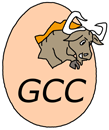# gcc

Implementation of programming language C

gcc is a short name for GNU C compiler, which is included in GNU Compiler Collection.

C was the first language of the Collection, and first version of its compiler was released in 1987. It is distributed under GNU GPL and is an important example of free software.GCC logo

## Examples:

### Factorial:

Example for versions gcc 3.4.5, gcc 3.4.5 (Objective-C), gcc 4.2.4, tcc 0.9.25

This example uses recursive factorial definition. Note that 13! and larger causes an overflow, so the last lines of the output look like this:

13! = 1932053504
14! = 1278945280
15! = 2004310016
16! = 2004189184

``````#include <stdio.h>

unsigned long long factorial(unsigned long long n)
{
if (n == 0) {
return 1;
} else {
return n * factorial (n - 1);
}
}

int main(void)
{
int n;
for (n = 0; n <= 16; n++) {
printf("%i! = %lld\n", n, factorial(n));
}
return 0;
}
``````

### CamelCase:

Example for versions gcc 3.4.5, gcc 4.2.4, tcc 0.9.25

This example is based on character-wise string processing. `fgets` here reads at most 99 characters into the string, and stops when it finds end-of-string character, so a long line might be split. C doesn’t provide boolean data type, so it has to be simulated using integer variable.

``````#include <stdio.h>

void main() {
char text,cc;
fgets(text, sizeof text, stdin);
int i,j=0,lastSpace=1;
for (i=0; text[i]!='\0'; i++)
if (text[i]>='A' && text[i]<='Z' || text[i]>='a' && text[i]<='z')
{   if (lastSpace>0)
cc[j] = toupper(text[i]);
else
cc[j] = tolower(text[i]);
j++;
lastSpace = 0;
}
else
lastSpace = 1;
cc[j]='\0';
printf("%s\n",cc);
}
``````

Example for versions gcc 4.2.4

This example requires C99, since it uses `complex` type introduced in it.

``````#include <stdio.h>
#include <complex.h>
#include <math.h>

void print(int ind, double complex x) {
printf("x%d = ", ind);
if (fabs(cimag(x)) < 1e-6)
printf("%f\n", creal(x));
else printf("(%f, %f)\n", creal(x), cimag(x));
}

int main() {
double A, B, C;
double D;
printf("A = ");
scanf("%lf", &A);
if (fabs(A)<1E-3) {
return 1;
}
printf("B = ");
scanf("%lf", &B);
printf("C = ");
scanf("%lf", &C);

A *= 2;
D = B*B-A*C*2.0;
if (fabs(D)<1E-3)
printf("x = %f", creal(-B/A));
else {
print(1, (-B+csqrt(D))/A);
print(2, (-B-csqrt(D))/A);
}
return 0;
}
``````

Example for versions Borland C++ Builder 6, Microsoft Visual C++ 9 (2008), g++ 3.4.5, gcc 3.4.5, gcc 3.4.5 (Objective-C), gcc 4.2.4, tcc 0.9.25

This example works both for C and C++, as well as for Objective-C which is superset of C.

``````#include <math.h>
#include <stdio.h>

int main()
{
int A, B, C;
double D;
printf("A = ");
scanf("%d", &A);
if (A == 0) {
return 0;
}

printf("B = ");
scanf("%d", &B);
printf("C = ");
scanf("%d", &C);

D = B * B - 4 * A * C;
if (D == 0) {
printf("x = %f\n", -B / 2.0 / A);
return 0;
}

if (D > 0) {
printf("x1 = %f\nx2 = %f\n",
(-B + sqrt(D)) / 2.0 / A, (-B - sqrt(D))/ 2.0 / A);
} else {
printf("x1 = (%f, %f)\nx2 = (%f, %f)\n",
-B / 2.0 / A, sqrt(-D) / 2.0 / A, -B / 2.0 / A, -sqrt(-D) / 2.0 /A);
}
return 0;
}
``````

### Hello, World!:

Example for versions Borland C++ Builder 6, Turbo C++ 1.01, g++ 3.4.5, gcc 3.4.5, gcc 3.4.5 (Objective-C), gcc 4.2.4, tcc 0.9.25
``````#include <stdio.h>

int main()
{
printf("Hello, World!\n");
return 0;
}
``````

### Fibonacci numbers:

Example for versions gcc 3.4.5, gcc 3.4.5 (Objective-C), tcc 0.9.25

This example uses recursive definition of Fibonacci numbers. Note the difference from C++ example: loop counter must be declared outside of the loop, and `printf` is used for output instead of `std::cout`.

``````#include <stdio.h>

int fibonacci(int n)
{
return ( n<=2 ? 1 : fibonacci(n-1) + fibonacci(n-2) );
}

int main(void)
{
int n;
for (n=1; n<=16; n++)
printf("%d, ", fibonacci(n));
printf("...\n");
return 0;
}
``````